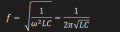# Frequency of ZVS driver changes through a transformer in LTspice

#### icantalktosneks

Joined Apr 23, 2021
35
I was simulating a ZVS driver in LTspice and in the first picture, the frequency is around 80kHz, which can also be calculated by the formula for resonant frequency.
In the second picture, the frequency changes to around 56kHz, even though all i added was the secondary coil for the transformer?
Am i missing something in LTspice, or is my theory wrong?

#### icantalktosneks

Joined Apr 23, 2021
35

#### ronsimpson

Joined Oct 7, 2019
2,259
even though all i added was the secondary coil for the transformer?
You changed more that adding a secondary.
Circuit 1, has L1 and L2 as independent coils.
Circuit 2, has L1 and L2 as a transformer. "K L1 L2 L4 1"
I think the "L4" is not the change you see but "K L1 L2 1" is the change you see.

RonS.

#### icantalktosneks

Joined Apr 23, 2021
35
You changed more that adding a secondary.
Circuit 1, has L1 and L2 as independent coils.
Circuit 2, has L1 and L2 as a transformer. "K L1 L2 L4 1"
I think the "L4" is not the change you see but "K L1 L2 1" is the change you see.

RonS.
Wait so how can I fix this?

#### ericgibbs

Joined Jan 29, 2010
16,018
hi ican,
The circuit is a self oscillating cross coupled circuit, any changes in L1 and L2 , also L4 values and the overall coupling Constant 'M' will effect and change the frequency of the oscillator.
Also the Load will effect the osc frequency.

L1 & L2 form part of the setting of the time constant [ freq] of the oscillator.
E

Last edited:

#### icantalktosneks

Joined Apr 23, 2021
35
hi ican,
The circuit is a self oscillating cross coupled circuit, any changes in L1 and L2 , also L4 values and the overall coupling Constant 'M' will effect and change the frequency of the oscillator.
Also the Load will effect the osc frequency.

L1 & L2 form part of the setting of the time constant [ freq] of the oscillator.
E
Just to be clear, in an actual circuit with a transformer with a center-tapped primary coil, can the frequency of the output voltage at the secondary coil be calculated by the following formula?With L representing the inductance of the primary coil and C representing the capacitance in parallel with the primary coil

#### ericgibbs

Joined Jan 29, 2010
16,018
hi,
Yes, the formula applies, providing that you know the inductances etc, is for fixed inductance & load condition.

What operating frequency do you calculate for just L1 and L2, no L4 secondary.??
Assuming a K coupling factor for L1 and L2 as 1.
E

Update:
Looking at your latest L1/L2 , you are showing no coupling between L1 and L2, how can that be possible if they are wound on the same former.?
That is why you measure around 80kHz
With K = 1 the freq is 56kHz.

Last edited:

#### icantalktosneks

Joined Apr 23, 2021
35
hi,
Yes, the formula applies, providing that you know the inductances etc, is for fixed inductance & load condition.

What operating frequency do you calculate for just L1 and L2, no L4 secondary.??
Assuming a K coupling factor for L1 and L2 as 1.
E

Update:
Looking at your latest L1/L2 , you are showing no coupling between L1 and L2, how can that be possible if they are wound on the same former.?
That is why you measure around 80kHz
With K = 1 the freq is 56kHz.
Ah i see, ok thank you so much once again

#### icantalktosneks

Joined Apr 23, 2021
35
hi,
Yes, the formula applies, providing that you know the inductances etc, is for fixed inductance & load condition.

What operating frequency do you calculate for just L1 and L2, no L4 secondary.??
Assuming a K coupling factor for L1 and L2 as 1.
E

Update:
Looking at your latest L1/L2 , you are showing no coupling between L1 and L2, how can that be possible if they are wound on the same former.?
That is why you measure around 80kHz
With K = 1 the freq is 56kHz.
Wait is the spice directive of "K L1 L2 L4 1" correct?
And if how is the value of 56kHz found, specifically, what would the value of L be?

#### icantalktosneks

Joined Apr 23, 2021
35

#### ericgibbs

Joined Jan 29, 2010
16,018
hi,
Yes about the extra 4uH.
What are you planning to use the project for.?

E

#### icantalktosneks

Joined Apr 23, 2021
35
hi,
Yes about the extra 4uH.
What are you planning to use the project for.?

E
I am trying to make an EMP generator, and i need a high voltage converter, hence the ZVS driver to step up the voltage from a DC power supply. A spark gap will be placed between its output and a coil to generate the EMP

#### Bordodynov

Joined May 20, 2015
2,995
The loop inductance of the first circuit is 2*L, and the second circuit is L*2^2=L*4. Therefore, 80/2^0.5 = 56.56854249492